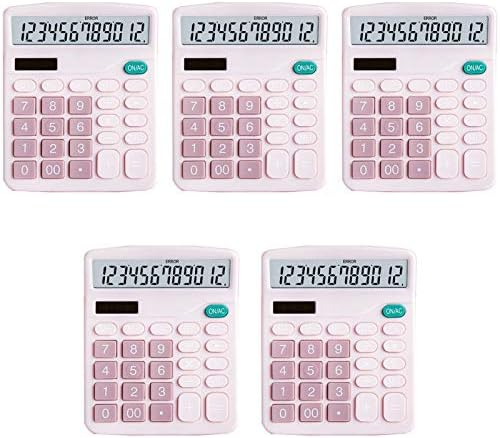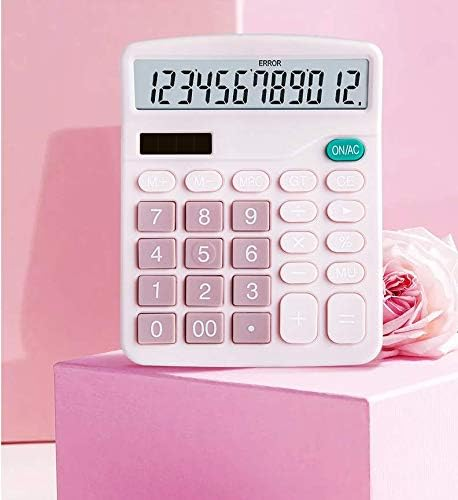As an Amazon Associate I earn from qualifying purchases

# YOUHO Calculator, 12-bit Solar Battery Dual Power Standard Function Electronic Calculator with Large LCD Display Office Calculator Black(5 Battery) (KK-837B, 5PACK，Pink)

\$19.99Price: \$19.99
(as of Apr 11,2021 00:39:58 UTC – Details)Color:Pink-12 Digits Splaks Electronic Calculator Instruction [M+] Adds the number shown on the display to the number stored in the memory Example:3*8 + 2*6. If you directly inputs these numbers to the calculator,you will see the wrong answer: 156. If you press [3*8], [M+], [2*6], [M+], then press [MRC] once, you will get the right answer: 36. [M-] Subtracts the number shown on the display to the number stored in the memory.
[MRC] Combination of [MR] and [MC].
[MR]: Recall the current memory register value.
[MC]: Clear the memory register (set to zero).
When you press the key once, the [MR] key was activated, when you press the key twice, the [MC] was activated. [GT] Whenever the [=] key has been pressed, the result will be stored into the Grand Total Memory. Example:If you want to calculate [2*3+3*5+6*8],press [2*3=], then [3*5=], then [6*8=]. Then press [GT], you will get the right answer  [MU] Stands for Mark Up, which is for calculating GROSS PROFIT MARGIN (GPM). Example: Enter  then press the [÷], then  then press the [MU] key, you will see 117.65 (15% of this total equates to 17.65) [Constant Calculations] To Calculate[5 * 5 * 5 * 5 + 2 + 2 = 629]. You Press[5 * === + 2 == ]. You will see  Note: If you have any question about the calculator, please contact us directly

## User Reviews

0.0 out of 5
0
0
0
0
0

There are no reviews yet.YOUHO Calculator, 12-bit Solar Battery Dual Power Standard Function Electronic Calculator with Large LCD Display Office Calculator Black(5 Battery) (KK-837B, 5PACK，Pink)

\$19.99Enable registration in settings - general
• Total (0)
0